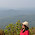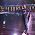## Friday, August 30, 2013

### Generate random 7 from random 5

Write a function to generate a random number between 1 and 7. You have been given a function that generates a random number between 1 and 5. The distribution between each of the numbers must be uniform.

1.This comment has been removed by the author.

2.int genRand1to7 ()
{
int i = genRand1to5();
int j = genRand1to5();
return (i+j)%8 ;
}

1.It's not uniform.

3.Let f() is retRand1to5() and g() is getRand1to7() then as per @AditiPal

g() = (f()+f())%8;

n = f();
g() = n+(log of n base sqrt(5));

Wouldn't it provide a more uniform distribution.

4.